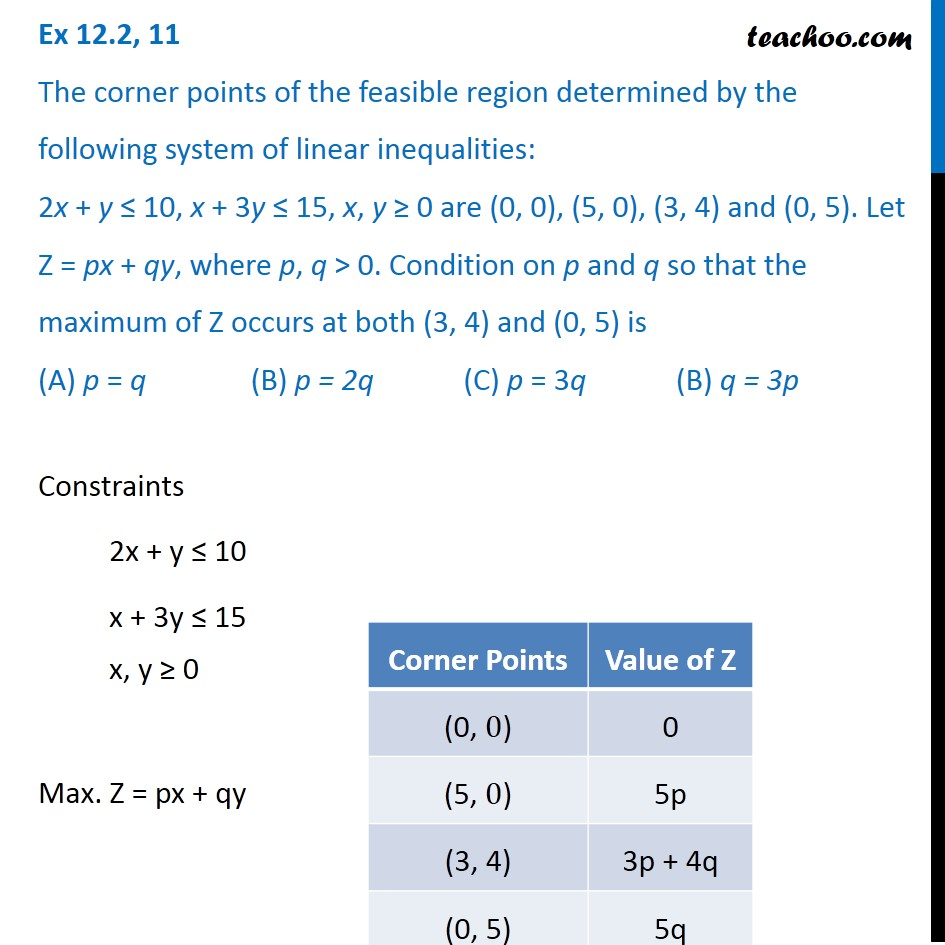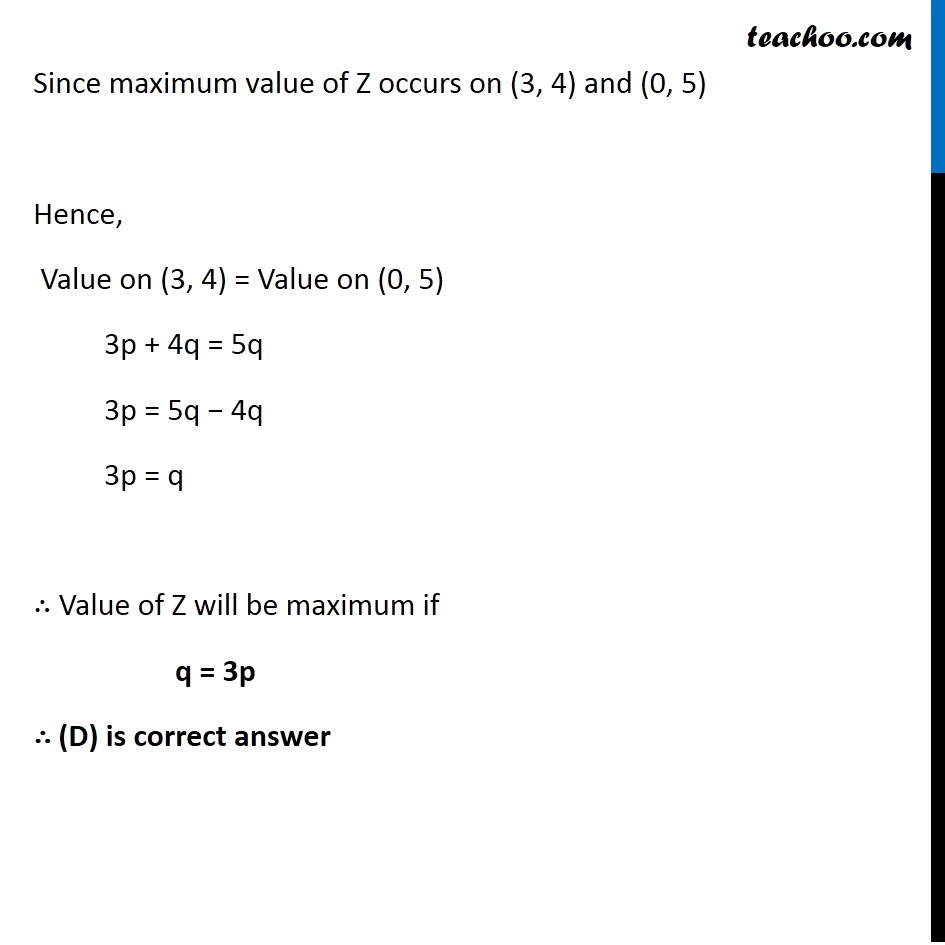Linear equations given - Bounded

Chapter 12 Class 12 Linear Programming
Concept wiseIntroducing your new favourite teacher - Teachoo Black, at only ₹83 per month

### Transcript

Ex 12.2, 11 The corner points of the feasible region determined by the following system of linear inequalities: 2x + y ≤ 10, x + 3y ≤ 15, x, y ≥ 0 are (0, 0), (5, 0), (3, 4) and (0, 5). Let Z = px + qy, where p, q > 0. Condition on p and q so that the maximum of Z occurs at both (3, 4) and (0, 5) is (A) p = q (B) p = 2q (C) p = 3q (B) q = 3p Constraints 2x + y ≤ 10 x + 3y ≤ 15 x, y ≥ 0 Max. Z = px + qy Since maximum value of Z occurs on (3, 4) and (0, 5) Hence, Value on (3, 4) = Value on (0, 5) 3p + 4q = 5q 3p = 5q − 4q 3p = q ∴ Value of Z will be maximum if q = 3p ∴ (D) is correct answer